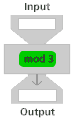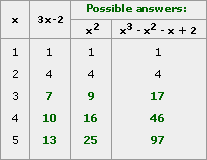# More Nonlinear Functions Homework

## Session 8, Homework

We said previously that algebra has become very much concerned with operations. So far, the only operations we’ve used are the ones from arithmetic. Let’s take a quick look at another kind of operation that often shows up in algebra. Consider the operation that divides a whole number by 3 and hands you back the remainder. This is usually called the “mod 3” operation.

Example: 17 divided by 3 is 5 with a remainder of 2, so we say 17 mod 3 = 2, or 17 = 2 (mod 3).Problem H1

If the input is 5, what is the output?

Problem H2

If the input is 12, what is the output?

Problem H3

If the input is 2, what is the output?

Problem H4

Now try some undoing:

• Describe all the numbers that produce an output of 1.
• What is the “pullback” of 2? (The pullback of an output is the collection of inputs that produce it.)
• What numbers produce an output of zero?
• How many possible outputs are there for this function? What are they?

Problem H5

Make an input/output table for this function. What kind of function is it?

#### Take It Further

Problem H6

Make a list of all the numbers that leave a remainder of 3 when divided by 5 and a remainder of 1 when divided by 3, then give one rule that would find them all.

#### Take It Further

Problem H7

If my age is divided by 3, the remainder is 2. If my age is divided by 5, the remainder is also 2. If my age is divided by 7, the remainder is 5. How old am I?

#### Take It Further

Problem H8

Here’s a table showing the first two outputs for the linear function y = 3x – 2. Come up with at least two other functions that match these outputs, and complete the table using your functions.

### Solutions

Problem H1

The output will be 2. Five goes into 3 once, and the remainder is 2.

Problem H2

The output will be 0. Twelve goes into 3 four times, with no remainder.

Problem H3

The output will be 2. Two doesn’t go into 3 at all, so the remainder is still 2.

Problem H4

1. There are an infinite number of answers, beginning with 1. The next is 4, then 7, then 10. Any number which is one more than a multiple of 3 produces an output of 1.
2. The “pullback” is all numbers which are two more than a multiple of 3: 2, 5, 8, 11, 14, … .
3. The numbers producing an output of zero will be all numbers which are zero more than a multiple of 3, or in other words, the multiples of 3: 0, 3, 6, 9, 12, … .
4. The only possible outputs are 0, 1, and 2. You might have noticed in H4(a)-H4(c) that every whole number falls into one of the three categories. Another explanation is that the only possible remainders when dividing by 3 are 0, 1, and 2.

Problem H5

It is a cyclic function. Here is a table:

 xy 00 1 1 2 2 3 0 4 1 5 2 6 0 7 1 8 2 9 0 10 1

Problem H6

This can be done by looking through the table of numbers which are one more than a multiple of 3 and looking for a pattern of those numbers which are also three more than a multiple of 5. The list consists of: 13, 28, 43, 58, 73, 88, …. The first of these numbers occurs with 13, and they repeat every 15 numbers. One rule to find all the numbers would be that they are all 13 more than a multiple of 15, or all numbers whose output is 13 in the “mod 15” operation.

Problem H7

This is harder to find, but the first two conditions mean that the age is two more than a multiple of 15 — those numbers are 2, 17, 32, 47, 62, 77, … . Among those numbers, the only one which is five more than a multiple of 7 is 47. There are other possible answers, but the others are greater than 100 (the first of these is 152). Overall, the condition is that the age must be equal to 47 (mod 105).

Problem H8

Here is one possibility for a completed table:Of course, there are lots of other possibilities!